Tel:400-803-9333
• Welcome to Microhm

#Position：Home » Technical Articles

# How to Calculate the Equivalent Resistance Value for Resistors in Circuit?

Writer：Microhm Page View：Date：2018-07-30

Resistors are often connected in series or parallel to create more complex networks. An example of 3 resistors in parallel is shown in the picture below.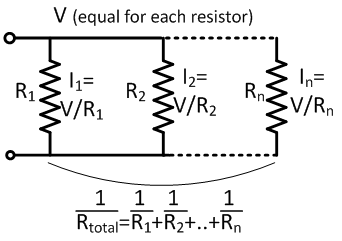The voltage across resistors in parallel is the same for each resistor. The current however, is in proportion to the resistance of each individual resistor. The equivalent resistance of several resistors in parallel is given by: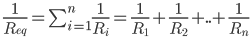The current through each resistor is given by: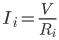A designer might for example combine several resistors with standard values  to reach a specific resistance value. For series connection, the current through each resistor is equal. There is only one path for the current to follow. The voltage drop however, is proportional to the resistance of each individual resistor.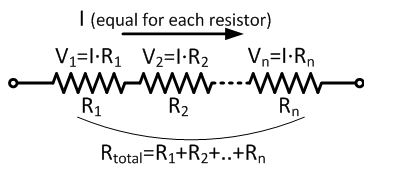The equivalent resistance of several resistors in series is given by: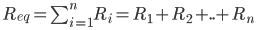The voltage across each resistor is calculated with Ohm’s law: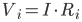Which resistor network the designer choose, it depends on the actual application.

Keywords：